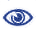# Prague Stringology Conference 2016

Fast Full Permuted Pattern Matching Algorithms on Multi-track Strings

 Abstract: A multi-track string is a tuple of strings of the same length. The full permuted pattern matching problem is, given two multi-track strings = (t1, t2, . . ., tN) and = (p1, p2, . . ., pN) such that |p1|= ⸳ ⸳ ⸳ =|pN| ≤ |t1|= ⸳ ⸳ ⸳ =|tN|, to find all positions i such that = (tr1[i:i+m-1], ⸳ ⸳ ⸳, trN[i:i+m-1]) for some permutation (r1, ⸳ ⸳ ⸳, rN) of (1, ⸳ ⸳ ⸳, N), where m=|p1| and \$t[i:j] denotes the substring of t from position i to j. We propose new algorithms that perform full permuted pattern matching practically fast. The first and second algorithms are based on the Boyer-Moore algorithm and the Horspool algorithm, respectively. The third algorithm is based on the Aho-Corasick algorithm where we use a multi-track character instead of a single character in the so-called goto function. The fourth algorithm is an improvement of the multi-track Knuth-Morris-Pratt algorithm that uses an automaton instead of the failure function of the original algorithm. Our experiment results demonstrate that those algorithms perform permuted pattern matching faster than existing algorithms.

 Download paper:PostScript PDF BibTeX reference
 Download presentation: